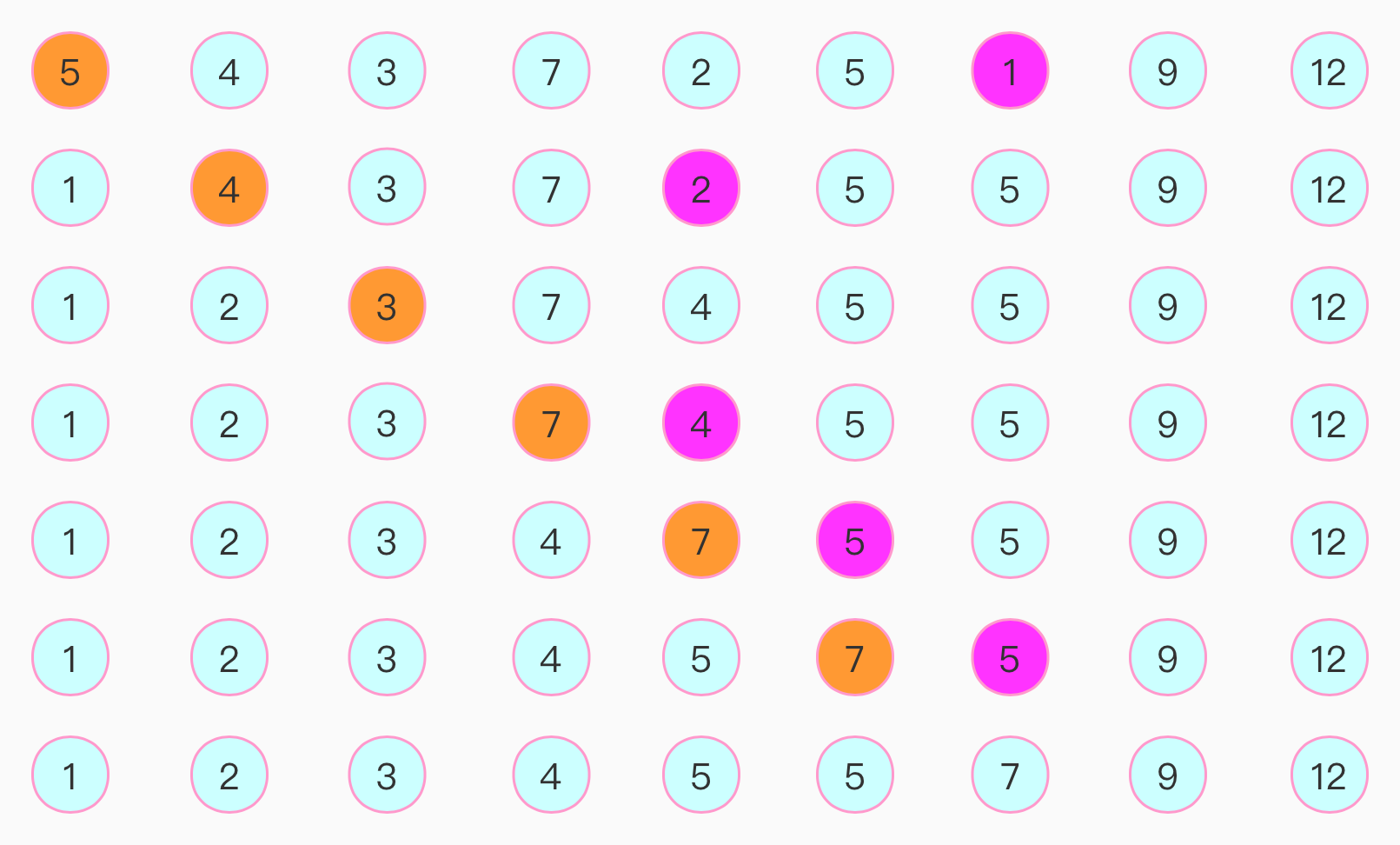# 算法-排序-选择排序

## 1.2 代码

``````class Untitled {
public static void insertSort(){
int[] numbers = {5,4,3,7,2,5,1,9,12};
int length = numbers.length;
for(int index = 0;index < length;index++){
//第一层循环找到需要比较的起始位置
int minValueIndex = index;
for(int index1 = index + 1;index1 < length; index1++){
//第二层循环从第一层循环的后一个元素开始比较
int tempValue = numbers[index1];
//只要后面的元素比前面的元素小，就记下这个元素的序号，一直找到最小的元素
if(tempValue < numbers[index]){
minValueIndex = index1;
}
}
//找到最小的元素序号之后，跟第一层循环中起始位置元素交换
int temp = numbers[index];
numbers[index] = numbers[minValueIndex];
numbers[minValueIndex] = temp;
}

for(int value:numbers){
System.out.println("value is:" + value);
}
}

public static void main(String[] args) {
insertSort();
}
}
``````

## 1.3 总结